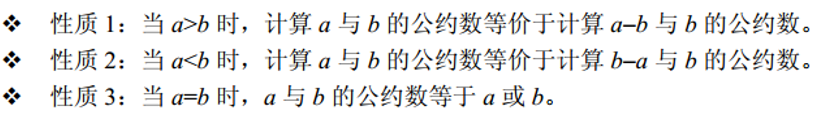• ## C语言求最小公倍数

千次阅读 2016-10-14 12:49:39
首先，需要知道一个公式，最小公倍数=两整数乘积/最大公...http://blog.csdn.net/qq_22497299/article/details/51922527 知道如何求最大公约数之后，只要知道公式，求最小公倍数就不在话下了。int lcm(int a, int b){
首先，需要知道一个公式，最小公倍数=两整数的乘积/最大公约数。
最大公约数通过辗转相除求得，具体求法可以看我的博客，利用位操作，实际上一行代码即可求出最大公约数。 http://blog.csdn.net/coder_panyy/article/details/52814945
知道如何求最大公约数之后，只要知道公式，求最小公倍数就不在话下了。

int lcm(int a, int b){
int temp1 = a,temp2 = b;
while(b^=a^=b^=a%=b);
return temp1*temp2/a;
}
展开全文• /*求最大公约数，最小公倍数的 */#include int maxdivisor(int ,int );/*求最大公约数函数*/int minmultiple(int ,int ,int );/*求最小公约数函数*/int dazaiqian(int *,int *);/*交换两个数，使大的在前的那个变量*...
/*求最大公约数，最小公倍数的 */#include int maxdivisor(int ,int );/*求最大公约数函数*/int minmultiple(int ,int ,int );/*求最小公约数函数*/int dazaiqian(int *,int *);/*交换两个数，使大的在前的那个变量*/int main (){int x,y,z,k;while(1){printf("inpute two numbers(larger than 0)\n");scanf("%d%d",&x,&y);if(x>=0&&y>=0){z=maxdivisor(x,y);k=minmultiple(x,y,z);printf("最大公约数是%d,最小公倍数是%d\n",z,k);break;}elseprintf("input wrong\n");}return 0;}int maxdivisor(int x,int y){int z;dazaiqian(&x,&y);z=x-y;while(z!=y){dazaiqian(&y,&z);x=y,y=z;z=x-y;}return z;}int dazaiqian(int *x,int *y){int z;if(*x{z=*x;*x=*y;*y=z;}}int minmultiple(int x,int y,int z){return x*y/z;}
展开全文• 求最小公倍数算法： 最小公倍数=两整数乘积÷最大公约数 求最大公约数算法： (1)辗转相除法 有两整数a和b： ① a%b得余数c ② 若c=0，则b即为两数最大公约数 ③ 若c≠0，则a=b，b=c，再回去执行① 例如求27和15...
求最小公倍数算法：
最小公倍数=两整数的乘积÷最大公约数
求最大公约数算法：
(1)辗转相除法
有两整数a和b：
① a%b得余数c
② 若c=0，则b即为两数的最大公约数
③ 若c≠0，则a=b，b=c，再回去执行①
例如求27和15的最大公约数过程为：
27÷15 余1215÷12余312÷3余0因此，3即为最大公约数
复制代码
1 #include<stdio.h>
2 int main()   /*  辗转相除法求最大公约数 /
3 {
4    int m, n, a, b, t, c;
5    printf(“Input two integer numbers:\n”);
6    scanf("%d%d", &a, &b);
7    m=a;   n=b;
8    while(b!=0)  / 余数不为0，继续相除，直到余数为0 /
9    { c=a%b; a=b;  b=c;}
10    printf(“The largest common divisor:%d\n”, a);
11    printf(“The least common multiple:%d\n”, mn/a);
12 }
复制代码
提供一种简写的方式：
1 int gcd(int a,int b)
2 {
3    return b==0?a:gcd(b,a%b);
4 }
⑵ 相减法
有两整数a和b：
① 若a>b，则a=a-b
② 若a<b，则b=b-a
③ 若a=b，则a（或b）即为两数的最大公约数
④ 若a≠b，则再回去执行①
例如求27和15的最大公约数过程为：
27－15＝12( 15>12 ) 15－12＝3( 12>3 )
12－3＝9( 9>3 ) 9－3＝6( 6>3 )
6－3＝3( 3==3 )
因此，3即为最大公约数
复制代码
1 #include<stdio.h>
2 int main ( )  /* 相减法求最大公约数 /
3 {
4    int m, n, a, b, c;
5    printf(“Input two integer numbers:\n”);
6    scanf ("%d,%d", &a, &b);m=a; n=b;
7      / a, b不相等，大数减小数，直到相等为止。/
8    while ( a!=b)
9          if (a>b)  a=a-b;
10          else  b=b-a;
11    printf(“The largest common divisor:%d\n”, a);
12    printf(“The least common multiple:%d\n”, mn/a);
13 }
复制代码
⑶穷举法
有两整数a和b：
① i=1
② 若a，b能同时被i整除，则t＝i
③ i++
④ 若 i <= a(或b)，则再回去执行②
⑤ 若 i > a(或b)，则t即为最大公约数，结束
改进：
① i= a(或b)
② 若a，b能同时被i整除，则i即为最大公约数，
结束
③ i–，再回去执行②
有两整数a和b：
① i=1
② 若a，b能同时被i整除，则t＝i
③ i++
④ 若 i <= a(或b)，则再回去执行②
⑤ 若 i > a(或b)，则t即为最大公约数，结束
改进：
① i= a(或b)
② 若a，b能同时被i整除，则i即为最大公约数，
结束
③ i–，再回去执行②
复制代码
1 #include<stdio.h>
2 int main ()  /* 穷举法求最大公约数 /
3 {
4    int  m, n, a, b, i, t;
5    printf(“Input two integer numbers:\n”);
6    scanf ("%d,%d", &a, &b);m=a;  n=b;
7    for (i=1; i<= a; i++)
8        if ( a%i == 0 && b%i ==0 )    t=i;
9    printf(“The largest common divisor:%d\n”, t);
10    printf(“The least common multiple:%d\n”, mn/t);
11 }
12 /*  改进后的
13    for (t= a; t>0; t-- )
14        if ( a%t == 0 && b%t ==0 )    break;
15 */
复制代码
复制代码
1 //穷举法求最小公倍数
2      for (i= a; ; i++ )
3          if ( i % a == 0 && i % b 0 )     break;
4      printf(“The least common multiple:%d\n”, i )
5
6 //多个数的最大公约数和最小公倍数
7      for (i= a; i>0; i-- )
8          if (a%i0&&b%i0&&c%i0)     break;
9      printf(“The largest common divisor:%d\n”, i);
10      for (i= a; ; i++ )
11          if (i%a0&&i%b0&&i% c==0)    break;
12      printf(“The least common multiple:%d\n”, i )


展开全文• 1.求最小公倍数 利用两个数都可以被其整除性质：最小公倍数可以从a倍数和b倍数中寻找，我们从大倍数中寻找效率较高。 2.求最大公约数 1.穷举法 两个数最大公约数必然是小于等于最小，故从...
1.求最小公倍数

利用两个数都可以被其整除的性质：最小公倍数可以从a的倍数和b的倍数中寻找，我们从大的数的倍数中寻找效率较高。

2.求最大公约数

1.穷举法

两个数的最大公约数必然是小于等于最小的数的，故从最小的数开始每次1开始寻找能同时整除两个数的，找到为止，即为最大公约数。

2.相减法

利用如下性质：3.欧几里得辗转相除法

完整的代码如下：

#include<stdio.h>
/*-----最小公倍数，最大公约数-----*/
/*求最小公倍数，利用两个数都可以被其整除的性质
最小公倍数可以从a的倍数和b的倍数中寻找，
我们从大的数的倍数中寻找效率较高
*/
int MinCommonMultiple(int a,int b){
int mcm,temp;
if(a>b){//使a<b
temp=a;
a=b;
b=temp;
}
mcm=b;//msm为最大的数
while(mcm%a!=0){
mcm+=b;//msm%b==0必成立
}
return mcm;
}
/*函数功能：使用穷举法，求最大公约数：
利用两个数都可以整除公约数的性质
*/
int MaxCommonFactor(int a,int b) {
int temp,mcf;
if(a>b){//使a<b
temp=a;
a=b;
b=temp;
}
mcf=a;//mcf是最小的
while(a%mcf!=0||b%mcf!=0){
mcf--;
}
return mcf;
}
/*函数功能：使用相减法，求正整数的最大公约数
函数返回最大公约数，-1表示没有最大公约数
*/
int MaxCommonFactor1(int a,int b){
if(a<=0||b<=0) {
return -1;
}
while(a!=b){
if(a>b){
a=a-b;
}
else if(b>a){
b=b-a;
}
}
return a;
}
/*函数功能:使用辗转相除法,求正整数的最大公约数
函数返回最大公约数，-1表示没有最大公约数
*/
int MaxCommonFactor2(int a,int b){
int r;
if(a<=0||b<=0) {
return -1;
}
do{
r=a%b;//即使a<b,最后也会交换过去使a>b
a=b;
b=r;
}while(r);//r!=0,程序结束时b=0;
return a;
}
int main(void){
int a,b,mcm,mcf,mcf1,mcf2;
scanf("%d %d",&a,&b);
mcm=MinCommonMultiple(a,b);
mcf=MaxCommonFactor(a,b);
mcf1=MaxCommonFactor1(a,b);
mcf2=MaxCommonFactor2(a,b);
printf("mcm=%d\n",mcm);
printf("mcf=%d\n",mcf);
printf("mcf1=%d\n",mcf1);
printf("mcf2=%d\n",mcf2);
return 0;
}


展开全文• 代码如下：例如27和15最大约数过程为：27÷15 余1215÷12余312÷3余0因此，3即为最大约数#include void main() /* 辗转相除法最大约数 */ {int m, n, a, b, t, c; printf("Input two integer numbers:\n...
• 参考代码：写不好看，但思路还是比较清晰，暂时也没出现bug#includevoid f14(int m,int n){int i=0;int num=1;int temp1=m,temp2=n;//用两个变量寄存m,n值int min=mfor(i=2;i<=min;i++){if((m%i==0)&&...
• C语言计算最小公倍数和最大公约数1..../*编写求最小公倍数的函数*/ int mul(int x,int y) { int z,i,t; if(x>y) { t=x; x=y; y=t; } for(i=x*y;i>=y;i--) { if((i%x==0)&&(i%数学
• C语言求最大公约数及最小公倍数 1. 最大公约数 1.1 定义 ​ 最大公约数（Greatest Common Divisor，GCD），也称最大公因数、最大公因子，是一种数学概念，指两个或多个整数共有约数中最大一个。 1.2 解法一：常规...
• 求最小公倍数算法： 最小公倍数=两整数乘积÷最大公约数 求最大公约数算法： (1)辗转相除法 有两整数a和b： ①a%b得余数c ②若c=0，则b即为两数最大公约数 ③ 若c≠0，则a=b，b=c，再回去执行① 例如求...
• int gcd(int a, int b){ // 最大公约数 return !b ? a : gcd(b, a%b); } int lcm(int a, int b){ //最小公倍数 return a/gcd(a,b)*b; }
• 数学决定了一个人编程的上限，这样说一点都不为过，接下来我将介绍一种公约数与公倍数的方法：辗转相除法。 算法流程： 结果处理：利用上面算法就可以出最大公约数，而最小公倍数=两数的乘积/最大公约数。 源...最大公约数 辗转相除法
• po上一段前几天在手机上写...但其实自己对于这段代码的也理解不透，涉及到了指针。今后自己再来解释这个问题吧。 方法？就是最粗暴列举罢了。虽然高中时学过啥辗转相除法，更相减损术，但还是没什么印象。。这就...
• 输入两个正整数，分别出最大公约数和最小公倍数 代码： #include&amp;amp;lt;stdio.h&amp;amp;gt; int main() { int m,n,a,b; printf(&amp;quot;输入两个正整数：&amp;quot;); scanf(&amp...
• C语言求两个数最大公约数和最小公倍数 解题思路：定义两个函数GCD（最大公约数 greatest common divisor）和LCM(最d小公倍数 least common multiple),然后进行调用即可。 在这里插入代码片 ...
• 例45：C语言编程实现两个数最大公约数和最小公倍数 解题思路：最大公因数，也称最大公约数、最大公因子，指两个或多个整数共有约数中最大一个；最小公倍数是指两个或多个整数公有倍数叫做它们公倍数，其中...程序设计
• 最大约数的法 首先了解它的一般法（欧几里得算法）：假设存在两个数A和B，假如A%B的结果不为0，那么A和B的最大约数是B与A%B的...最大约数的代码：（基于C++实现的函数） int gcd(int a,int b) { in...
• c语言最基本一些小程序源代码： 方法一： #include int main() { int data1,data2,i;... printf("please input two datas:\n");... if(data1>data2)// 最小公倍数； { for(i=data1;i*dataLinux
• 输入两个正整数m和n，求其最大公约数和最小公倍数。 输入 两个整数 输出 最大公约数，最小公倍数 样例输入 ...用两数相乘再除以最大公因数来求最小公倍数代码： int multiple(int x,int y) //...
• 题目描述：在一个程序里用C语言求出用户输入来两个数最大公约数和最小公倍数。 例如用户输入为 7 9 则输出应为 1 63 代码如下 #include<stdio.h>...//求最小公倍数 int main() { int a...
• 最大约数有多种方法，常见有质因数分解法、短除法、辗转相除法、更相减损法。 在C语言里，我们常用辗转相除法和更相减损法来写；但我们通过计算机计算能力也可以使用暴力穷举法！ 一、暴力穷举法： 我们先...
• 相信大家对最大公约数和最小公倍数一定不会陌生吧,那么在这里我就不做太多解释了,直接上代码: 方法1: #include <stdio.h> int hf(int u , int v) { int t , r; if(v>u){ t=u;u=v;v=t; } while((r = u%v)...
• Today，让我来带大家学习一下如何用C语言写出两个数的最大公约数和最小公倍数的代码，这个方法不仅在C语言中有用，其他语言都可以用的。 首先，来看看怎么最大公约数，最大公约数需要用到欧几里得算法，也...
• 输入两个正整数 m 和 n ,其最大公因数与最小公倍数？ 使用C语言编写 代码. // An highlighted block #include<stdio.h> int main(void) { int m, n, r, m2, n2; int MaxCom, MinCom; scanf("%d%d", &...
• 这里写自定义目录标题欢迎使用Markdown编辑器新的改变功能快捷键合理的创建标题，有助于目录的生成如何改变文本的样式插入链接与图片如何插入一段漂亮的代码片生成一个适合你的列表创建一个表格设定内容居中、居左、...程序设计
• 以下是我的代码，提交不通过（显示wrong answer），是这种方法不行吗？ ![图片说明](https://img-ask.csdn.net/upload/201701/25/1485275842_61136.png) ` #include int main(int argc, char* argv[]) { ...c...

# c语言求最小公倍数的代码c语言 订阅